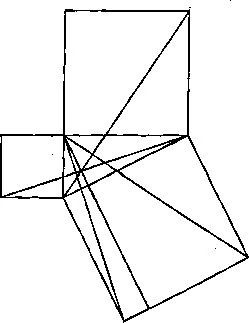316 PagesProof of the Adrakhonic Theorem as seen on the hull of the Geometer's ship the Daban Urnud

Mathic name for the Pythagorean Theorem. A diagram for a proof of this theorem can be seen on the outside of the Daban Urnud. This is seen as a declaration that the occupants are Theors of some sort.

The DU displays a visual proof of the Pythagorean Theorem that is unusual, but not wholly unintelligible. Whereas two popular proofs   rely on the juxtaposition of similar shapes, and one  relies on the projection of rectangles and parallelograms of equal bases, the DU proof perhaps demonstrates a basic knowledge of trigonometry.

More than one proof can be based on the DU diagram. One of them is the proof from Euclid's Elements. Another uses the formula for area of a right triangle and that the the two other triangles each appear twice after rotation by a right angle so each copy has the same area. One gets a system of equations. Eliminating the areas of the two triangles that each appear twice and simplifying yields the Pythagorean formula.

Community content is available under CC-BY-SA unless otherwise noted.